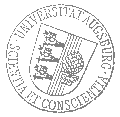# Universität AugsburgInstitut für MathematikAnalysis-Seminar Augsburg-München

Professor Dr. Carsten Hartmann
Freie Universität Berlin

spricht am

Donnerstag, 19. April 2018

um

16:30 Uhr

im

Raum 2004 (L1)

über das Thema:

## »Duality of estimation and control and its application to rare event simulation«

 Abstract: The talk will be devoted to the question of computing the optimal change of measure for certain classes of rare event simulation problems that appear in statistical mechanics, e.g. in molecular dynamics. The method is based on a representation of the rare event sampling problem as an equivalent (or: dual) stochastic optimal control problem, whose value function characterizes the optimal (i.e. minimum variance) change of measure. The specific duality behind the problem is then used to devise numerical algorithms for computing the optimal change of measure. I will describe two approaches in some detail that are built on a semi-parametric representation of the value function: a cross-entropy based stochastic approximation algorithm and a Monte-Carlo based least-squares discretisation of a related forward-backward stochastic differential equation. I will discuss the general approach, with a particular focus on the choice of the ansatz functions and the solution of high-dimensional problems, and illustrate the numerical method with simple toy examples.

 Hierzu ergeht herzliche Einladung. Prof. Dr. Bernd Schmidt

Kaffee, Tee und Gebäck eine halbe Stunde vor Vortragsbeginn im Raum 2006 (L1).

[Impressum]      [Datenschutz]      wwwadm@math.uni-augsburg.de,    Mo 19-Mär-2018 08:52:39 MESZ